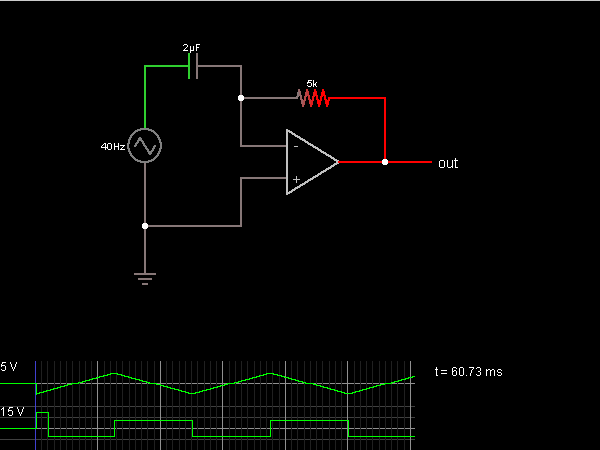# Circuit Simulator - Differentiator (inverting)

Differentiator (inverting)Circuit Description:
This circuit calculates the derivative of the input, meaning that the output voltage is proportional to the (negative) rate of change of the input voltage.

The voltage across the capacitor is proportional to the charge stored in it (the integral of the current). The op-amp attempts to keep its – input at the same voltage as the + input (which is at ground), so the voltage drop across the capacitor must always be equal to the input voltage. This requires the op-amp to have a negative voltage when the input voltage is rising (to drain the capacitor to compensate), and a positive voltage when the input voltage is falling. If the input voltage changes too fast, the op-amp will hit its upper or lower limit and so it won't be able to keep the – input at ground all the time.

Discussion:
1 comments Page 1 of 1.

Vinod gurjar said:   8 years ago
Very helpful site for the students who are preparing competitive engineering exam.
(5)